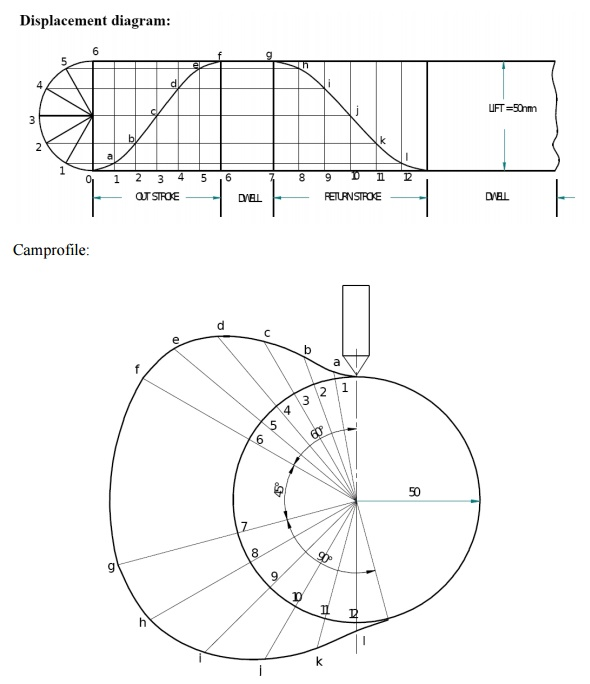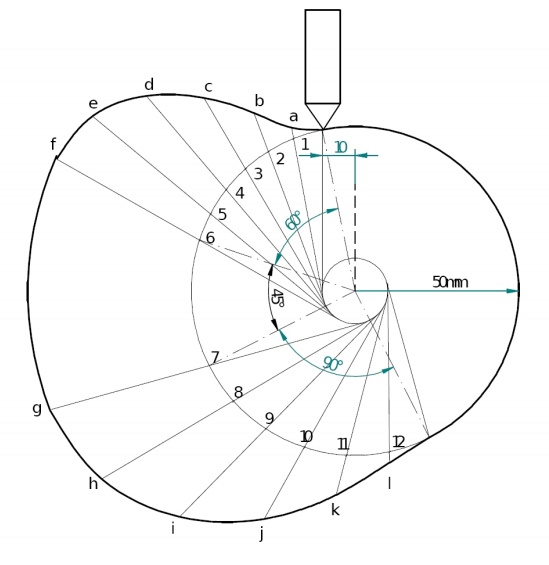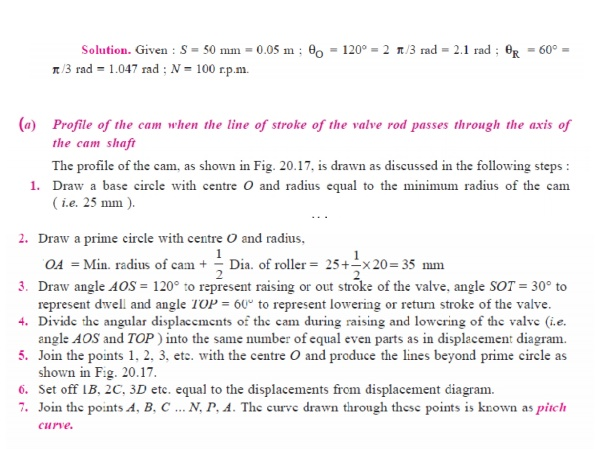Home | | Kinematics of Machinery | Kinematics of Cam Mechanisms Cams

# Kinematics of Cam Mechanisms Cams

1 Introduction 1.1 Types of cams 2 Types of follower motion 3 Displacement diagrams 4 Draw the cam profile for following conditions 5 Layout of plate cam profiles 6 Derivatives of Follower motion 7 Circular arc and Tangent cams 8 Pressure angle and undercutting

KINEMATICS OF CAM MECHANISMS CAMS

1 INTRODUCTION

A cam is a mechanical device used to transmit motion to a follower by direct contact. The driver is called the cam and the driven member is called the follower. In a cam follower pair, the cam normally rotates while the follower may translate or oscillate. A familiar example is the camshaft of an automobile engine, where the cams drive the push rods (the followers) to open and close the valves in synchronization with the motion of the pistons.Types of cams

Cams can be classified based on their physical shape.

a) Disk or plate cam (Fig. 6.1a and b): The disk (or plate) cam has an irregular contour to impart a specific motion to the follower. The follower moves in a plane perpendicular to the axis of rotation of the camshaft and is held in contact with the cam by springs or gravity.Fig. 6.1 Plate or disk cam.

b) Cylindrical cam (Fig. 6.2): The cylindrical cam has a groove cut along its cylindrical surface. The roller follows the groove, and the follower moves in a plane parallel to the axis of rotation of the cylinder.Fig. 6.2 Cylindrical cam.

c) Translating cam (Fig. 6.3a and b). The translating cam is a contoured or grooved plate sliding on a guiding surface(s). The follower may oscillate (Fig. 6.3a) or reciprocate (Fig. 6.3b). The contour or the shape of the groove is determined by the specified motion of the follower.Fig. 6.3 Translating cam

Types of followers:

(i) Based on surface in contact. (Fig.6.4)

(a)  Knife edge follower

(b)  Roller follower

(c)Flat faced follower

(d)  Spherical followerFig. 6.4  Types of followers

(ii)Based on type of motion: (Fig.6.5)

(a) Oscillating follower

(b) Translating follower(iii)           Based on line of motion:

(a) Radial follower: The lines of movement of in-line cam followers pass through the centers of the camshafts (Fig. 6.4a, b, c, and d).

(b) Off-set follower: For this type, the lines of movement are offset from the centers of the camshafts (Fig. 6.6a, b, c, and d).Fig.6.6 Off set followers

Cam nomenclature (Fig. 6.7):Cam Profile  The contour of the working surface of the cam.

Tracer Point The point at the knife edge of a follower, or the center of a roller, or the center of a spherical face.

Pitch Curve  The path of the tracer point.

Base Circle The smallest circle drawn, tangential to the cam profile, with its center on the axis of the camshaft. The size of the base circle determines the size of the cam.

Prime Circle The smallest circle drawn, tangential to the pitch curve, with its center on the axis of the camshaft.

Pressure Angle The angle between the normal to the pitch curve and the direction of motion of the follower at the point of contact.

2 Types of follower motion:

Cam follower systems are designed to achieve a desired oscillatory motion. Appropriate displacement patterns are to be selected for this purpose, before designing the cam surface. The cam is assumed to rotate at a constant speed and the follower raises, dwells, returns to its original position and dwells again through specified angles of rotation of the cam, during each revolution of the cam. Some of the standard follower motions are as follows:

They are, follower motion with,

(c) Uniform velocity

(b) Modified uniform velocity

(c)  Uniform acceleration and deceleration

(d) Simple harmonic motion

(e)  Cycloidal motion

3 Displacement diagrams:

Displacement diagrams: In a cam follower system, the motion of the follower is very important. Its displacement can be plotted against the angular displacement θ of the cam and it is called as the displacement diagram. The displacement of the follower is plotted along the y-axis and angular displacement θ of the cam is plotted along x-axis. From the displacement diagram, velocity and acceleration of the follower can also be plotted for different angular displacements θ of the cam. The displacement, velocity and acceleration diagrams are plotted for one cycle of operation i.e., one rotation of the cam. Displacement diagrams are basic requirements for the construction of cam profiles. Construction of displacement diagrams and calculation of velocities and accelerations of followers with different types of motions are discussed in the following sections.

(a) Follower motion with Uniform velocity:

Fig.3.8 shows the displacement, velocity and acceleration patterns of a follower having uniform velocity type of motion. Since the follower moves with constant velocity, during rise and fall, the displacement varies linearly with θ. Also, since the velocity changes from zero to a finite value, within no time, theoretically, the acceleration becomes infinite at the beginning and end of rise and fall.It is observed in the displacement diagrams of the follower with uniform velocity that the acceleration of the follower becomes infinite at the beginning and ending of rise and return strokes. In order to prevent this, the displacement diagrams are slightly modified.In the modified form, the velocity of the follower changes uniformly during the beginning and end of each stroke. Accordingly, the displacement of the follower varies parabolic ally during these periods. With this modification, the acceleration becomes constant during these periods, instead of being infinite as in the uniform velocity type of motion. The displacement, velocity and acceleration patterns shown in fig 3.9acceleration and retardation (UARM):

Here, the displacement of the follower varies parabolically with respect to angular displacement of cam. Accordingly, the velocity of the follower varies uniformly with respect to angular displacement of cam. The acceleration/retardation of the follower becomes constant accordingly. The displacement, velocity and acceleration patterns are shown fig 3.10(d) Simple Harmonic Motion: In fig3.11, the motion executed by point Pl, which is the projection of point P on the vertical diameter is called simple harmonic motion. Here, P moves with uniform angular velocity ωp, along a circle of radius r (r = s/2).Cycloidal motion:

Cycloid is the path generated by a point on the circumference of a circle, as the circle rolls without slipping, on a straight/flat surface. The motion executed by the follower here, is similar to that of the projection of a point moving along a cyloidal curve on a vertical line as shown in figure 6.12.(1) Draw the cam profile for following conditions:

Follower type = Knife edged, in-line; lift = 50mm; base circle radius = 50mm; out stroke with SHM, for 600 cam rotation; dwell for 450cam rotation; return stroke with SHM, for 90ocam rotation; dwell for the remaining period.

(2) Draw the cam profile for the same operating conditions of with the follower off set by 10 mm to the left of cam center.Cam profile with 10 mm offset:(2) Draw the cam profile for following conditions:

Follower type = roller follower, in-line; lift = 25mm; base circle radius = 20mm; roller radius = 5mm; out stroke with Uniform acceleration and retardation, for 1200 cam rotation; dwell for 600 cam rotation; return stroke with Uniform acceleration and retardation , for 900 cam rotation; dwell for the remaining period.

(4) Draw the cam profile for conditions same with follower off set to right of cam center by 5mm and cam rotating counter clockwise.(3) Draw the cam profile for following conditions:

Follower type = knife edged follower, in line; lift = 30mm; base circle radius = 20mm;out stroke with uniform velocity in 1200 of cam rotation; dwell for 600; return stroke with uniform velocity, during 900 of cam rotation; dwell for the remaining period.

Displacement Diagram(4) Draw the cam profile for following conditions:

Follower type = flat faced follower, in line; follower rises by 20mm with SHM in 1200of cam rotation, dwells for 300 of cam rotation; returns with SHM in 1200 of cam rotation and dwells during the remaining period. Base circle radius = 25mm.

Displacement Diagram:5 Layout of plate cam profiles:

·        Drawing the displacement diagrams for the different kinds of the motions and the plate cam profiles for these different motions and different followers.

·        SHM, Uniform velocity, Uniform acceleration and retardation and Cycloidal motions

·        Knife-edge, Roller, Flat-faced and Mushroom followers.

6Derivatives of Follower motion:

·        Velocity and acceleration of the followers for various types of motions.

·        Calculation of Velocity and acceleration of the followers for various types of motions.

7Circular arc and Tangent cam s:

·        Circular arc

·        Tangent cam

Standard cam motion:

·        Simple Harmonic Motion

·        Uniform velocity motion

·        Uniform acceleration and retardation motion

·        Cycloidal motion

8Pressure angle and undercutting:

·        Pressure angle

·        UndercuttingStudy Material, Lecturing Notes, Assignment, Reference, Wiki description explanation, brief detail
Mechanical : Kinematics of Machinery : Kinematics of Cam Mechanisms Cams : Kinematics of Cam Mechanisms Cams |